# 10.2 Non-right triangles: law of cosines  (Page 5/8)

 Page 5 / 8

Explain the relationship between the Pythagorean Theorem and the Law of Cosines.

When must you use the Law of Cosines instead of the Pythagorean Theorem?

The Law of Cosines must be used for any oblique (non-right) triangle.

## Algebraic

For the following exercises, assume $\text{\hspace{0.17em}}\alpha \text{\hspace{0.17em}}$ is opposite side $\text{\hspace{0.17em}}a,\beta \text{\hspace{0.17em}}$ is opposite side $\text{\hspace{0.17em}}b,\text{\hspace{0.17em}}$ and $\text{\hspace{0.17em}}\gamma \text{\hspace{0.17em}}$ is opposite side $\text{\hspace{0.17em}}c.\text{\hspace{0.17em}}$ If possible, solve each triangle for the unknown side. Round to the nearest tenth.

$\gamma =41.2°,a=2.49,b=3.13$

$\alpha =120°,b=6,c=7$

11.3

$\beta =58.7°,a=10.6,c=15.7$

$\gamma =115°,a=18,b=23$

34.7

$\alpha =119°,a=26,b=14$

$\gamma =113°,b=10,c=32$

26.7

$\beta =67°,a=49,b=38$

$\alpha =43.1°,a=184.2,b=242.8$

257.4

$\alpha =36.6°,a=186.2,b=242.2$

$\beta =50°,a=105,b=45{}_{}{}^{}$

not possible

For the following exercises, use the Law of Cosines to solve for the missing angle of the oblique triangle. Round to the nearest tenth.

$\text{\hspace{0.17em}}a=42,b=19,c=30;\text{\hspace{0.17em}}$ find angle $\text{\hspace{0.17em}}A.$

find angle $\text{\hspace{0.17em}}C.$

95.5°

$\text{\hspace{0.17em}}a=16,b=31,c=20;\text{\hspace{0.17em}}$ find angle $\text{\hspace{0.17em}}B.$

$\text{\hspace{0.17em}}a=13,\text{\hspace{0.17em}}b=22,\text{\hspace{0.17em}}c=28;\text{\hspace{0.17em}}$ find angle $\text{\hspace{0.17em}}A.$

26.9°

$a=108,\text{\hspace{0.17em}}b=132,\text{\hspace{0.17em}}c=160;\text{\hspace{0.17em}}$ find angle $\text{\hspace{0.17em}}C.\text{\hspace{0.17em}}$

For the following exercises, solve the triangle. Round to the nearest tenth.

$A=35°,b=8,c=11$

$B\approx 45.9°,C\approx 99.1°,a\approx 6.4$

$B=88°,a=4.4,c=5.2$

$C=121°,a=21,b=37$

$A\approx 20.6°,B\approx 38.4°,c\approx 51.1$

$a=13,b=11,c=15$

$a=3.1,b=3.5,c=5$

$A\approx 37.8°,B\approx 43.8,C\approx 98.4°$

$a=51,b=25,c=29$

For the following exercises, use Heron’s formula to find the area of the triangle. Round to the nearest hundredth.

Find the area of a triangle with sides of length 18 in, 21 in, and 32 in. Round to the nearest tenth.

177.56 in 2

Find the area of a triangle with sides of length 20 cm, 26 cm, and 37 cm. Round to the nearest tenth.

$a=\frac{1}{2}\text{\hspace{0.17em}}\text{m},b=\frac{1}{3}\text{\hspace{0.17em}}\text{m},c=\frac{1}{4}\text{\hspace{0.17em}}\text{m}$

0.04 m 2

0.91 yd 2

## Graphical

For the following exercises, find the length of side $x.$ Round to the nearest tenth.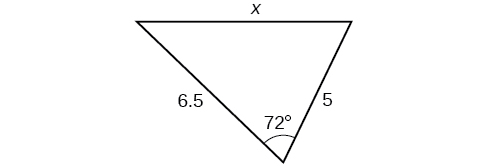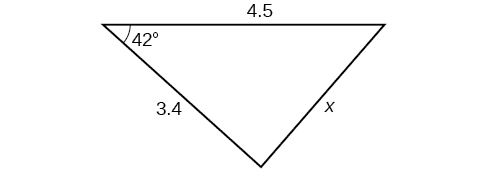3.0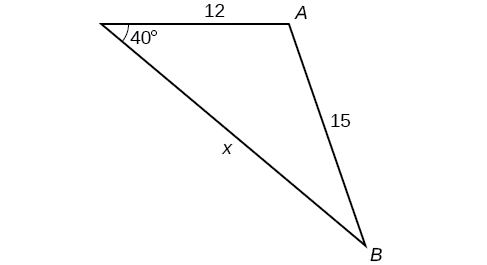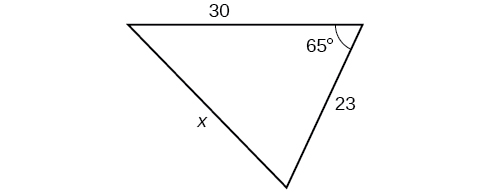29.1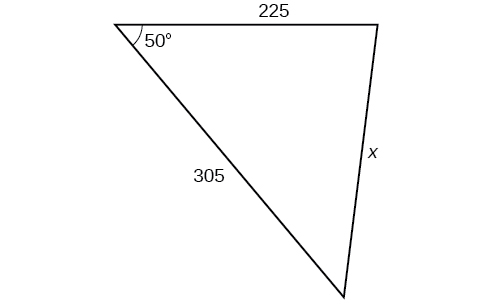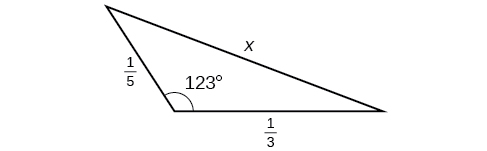0.5

For the following exercises, find the measurement of angle $\text{\hspace{0.17em}}A.$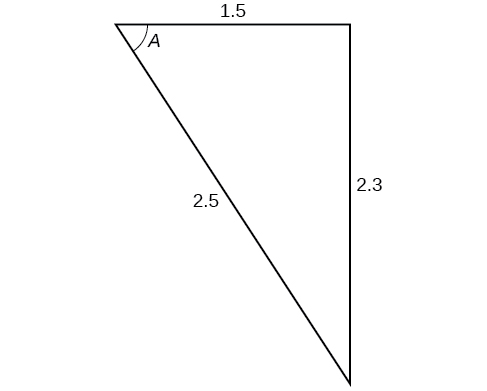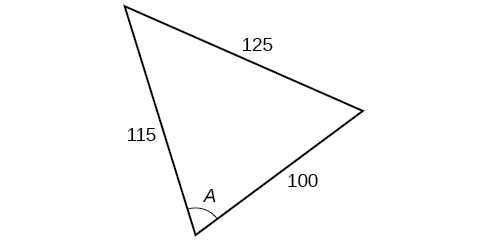70.7°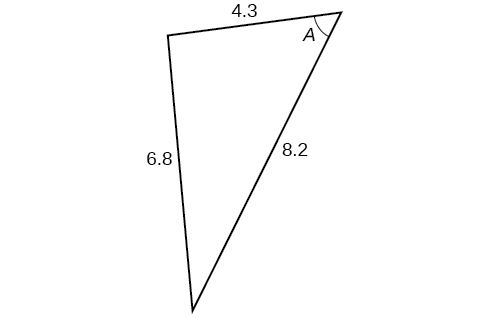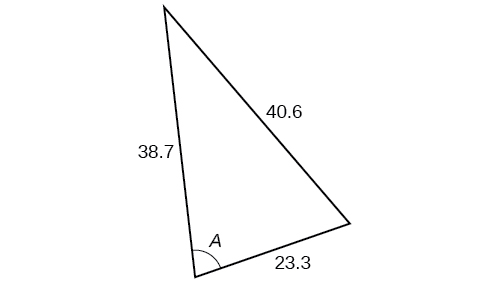77.4°

Find the measure of each angle in the triangle shown in [link] . Round to the nearest tenth.

For the following exercises, solve for the unknown side. Round to the nearest tenth.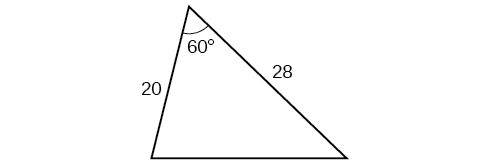25.0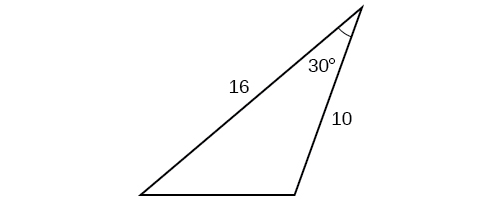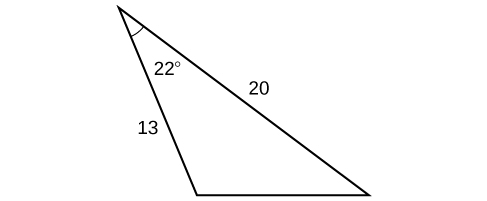9.3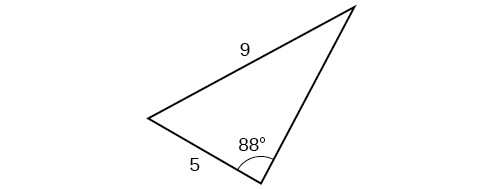For the following exercises, find the area of the triangle. Round to the nearest hundredth.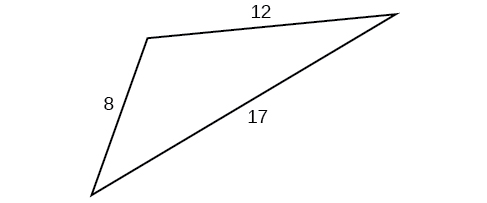43.52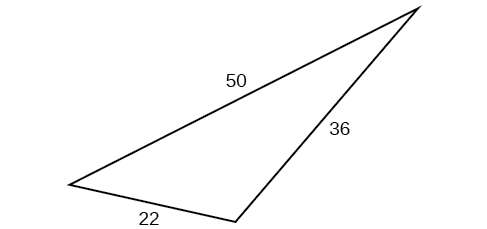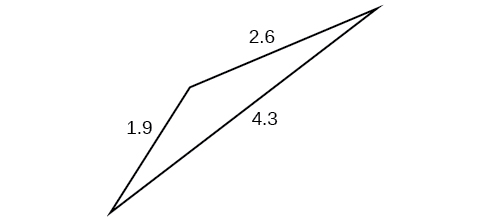1.41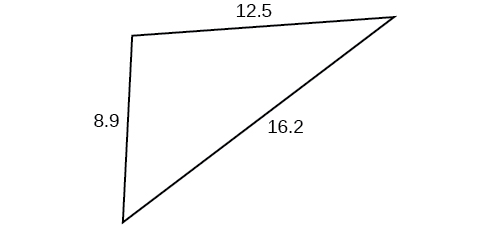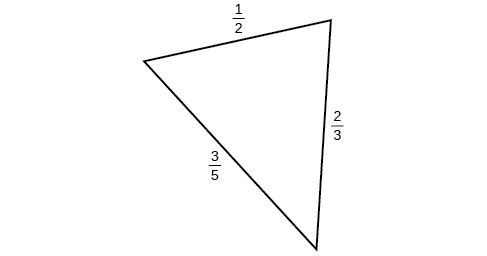0.14

## Extensions

A parallelogram has sides of length 16 units and 10 units. The shorter diagonal is 12 units. Find the measure of the longer diagonal.

The sides of a parallelogram are 11 feet and 17 feet. The longer diagonal is 22 feet. Find the length of the shorter diagonal.

18.3

The sides of a parallelogram are 28 centimeters and 40 centimeters. The measure of the larger angle is 100°. Find the length of the shorter diagonal.

A regular octagon is inscribed in a circle with a radius of 8 inches. (See [link] .) Find the perimeter of the octagon.

48.98

A regular pentagon is inscribed in a circle of radius 12 cm. (See [link] .) Find the perimeter of the pentagon. Round to the nearest tenth of a centimeter.

For the following exercises, suppose that $\text{\hspace{0.17em}}{x}^{2}=25+36-60\mathrm{cos}\left(52\right)\text{\hspace{0.17em}}$ represents the relationship of three sides of a triangle and the cosine of an angle.

Draw the triangle.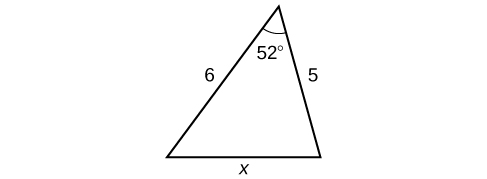Find the length of the third side.

For the following exercises, find the area of the triangle.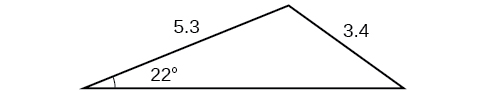7.62

#### Questions & Answers

The sequence is {1,-1,1-1.....} has
amit Reply
circular region of radious
Kainat Reply
how can we solve this problem
Joel Reply
Sin(A+B) = sinBcosA+cosBsinA
Eseka Reply
Prove it
Eseka
Please prove it
Eseka
hi
Joel
June needs 45 gallons of punch. 2 different coolers. Bigger cooler is 5 times as large as smaller cooler. How many gallons in each cooler?
Arleathia Reply
find the sum of 28th term of the AP 3+10+17+---------
Prince Reply
I think you should say "28 terms" instead of "28th term"
Vedant
if sequence sn is a such that sn>0 for all n and lim sn=0than prove that lim (s1 s2............ sn) ke hole power n =n
SANDESH Reply
write down the polynomial function with root 1/3,2,-3 with solution
Gift Reply
if A and B are subspaces of V prove that (A+B)/B=A/(A-B)
Pream Reply
write down the value of each of the following in surd form a)cos(-65°) b)sin(-180°)c)tan(225°)d)tan(135°)
Oroke Reply
Prove that (sinA/1-cosA - 1-cosA/sinA) (cosA/1-sinA - 1-sinA/cosA) = 4
kiruba Reply
what is the answer to dividing negative index
Morosi Reply
In a triangle ABC prove that. (b+c)cosA+(c+a)cosB+(a+b)cisC=a+b+c.
Shivam Reply
give me the waec 2019 questions
Aaron Reply
the polar co-ordinate of the point (-1, -1)
Sumit Reply

### Read also:

#### Get the best Algebra and trigonometry course in your pocket!

Source:  OpenStax, Algebra and trigonometry. OpenStax CNX. Nov 14, 2016 Download for free at https://legacy.cnx.org/content/col11758/1.6
Google Play and the Google Play logo are trademarks of Google Inc.

Notification Switch

Would you like to follow the 'Algebra and trigonometry' conversation and receive update notifications?ByByByByByByBy Dravida Mahadeo-J...